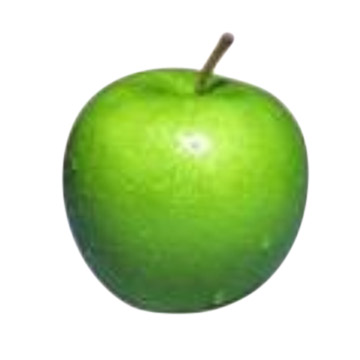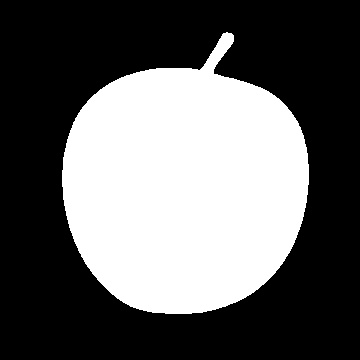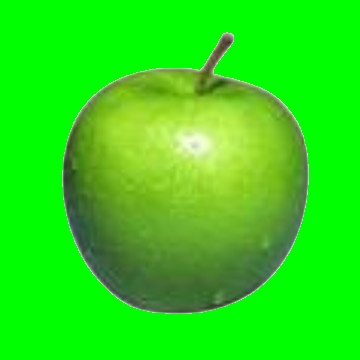# Extracting Contours with OpenCV

Yesterday I was asked how to extract a contour from a given image in OpenCV. Here is an example. Imagine we got this tasty apple and we want to put it in another image (with a green background):One solution is to first detect the edges of the apple with a Canny filter, then find the contours with OpenCV's findContours and create a mask with drawContours:Finally copy the masked original image to the new image, which means only the areas of the contours will be copied... and you are done. You end up with a tasty apple on a green background:In code this translates to:

``````#include "cv.h"
#include "highgui.h"

using namespace cv;
using namespace std;

int main() {

// read in the apple (change path to the file)

Mat img1;
cvtColor(img0, img1, CV_RGB2GRAY);

Canny(img1, img1, 100, 200);

// find the contours
vector< vector<Point> > contours;
findContours(img1, contours, CV_RETR_EXTERNAL, CV_CHAIN_APPROX_NONE);

// you could also reuse img1 here
Mat mask = Mat::zeros(img1.rows, img1.cols, CV_8UC1);

// CV_FILLED fills the connected components found

/*
Before drawing all contours you could also decide
to only draw the contour of the largest connected component
found. Here's some commented out code how to do that:
*/

//    vector<double> areas(contours.size());
//    for(int i = 0; i < contours.size(); i++)
//        areas[i] = contourArea(Mat(contours[i]));
//    double max;
//    Point maxPosition;
//    minMaxLoc(Mat(areas),0,&max,0,&maxPosition);
//    drawContours(mask, contours, maxPosition.y, Scalar(1), CV_FILLED);

// let's create a new image now
Mat crop(img0.rows, img0.cols, CV_8UC3);

// set background to green
crop.setTo(Scalar(0,255,0));

// and copy the magic apple

// normalize so imwrite(...)/imshow(...) shows the mask correctly!

// show the images
imshow("original", img0);